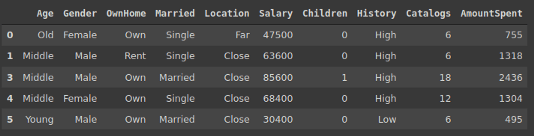## Cleaner Data Analysis with Pandas Using Pipes

[ad_1]

By Soner Yıldırım, Data Science EnthusiastPandas is a widely-used data analysis and manipulation library for Python. It provides numerous functions and methods to provide robust and efficient data analysis process.

In a typical data analysis or cleaning process, we are likely to perform many operations. As the number of operations increase, the code starts to look messy and harder to maintain.

One way to overcome this issue is using the pipe function of Pandas. What pipe function does is to allow combining many operations in a chain-like fashion.

In this article, we will go over examples to understand how the pipe function can be used to produce cleaner and more maintainable code.

We will first do some data cleaning and manipulation on a sample dataframe in separate steps. After that, we will combine these steps using the pipe function.

Let’s start by importing libraries and creating the dataframe.

``````import numpy as np
import pandas as pdmarketing = pd.read_csv("/content/DirectMarketing.csv")
marketing.head()``````(image by author)

The dataset contains information about a marketing campaign. It is available here on Kaggle.

The first operation I want to do is to drop columns that have lots of missing values.

``````thresh = len(marketing) * 0.6
marketing.dropna(axis=1, thresh=thresh, inplace=True)``````

The code above drops the columns with 40 percent or more missing values. The value we pass to the thresh parameter of dropna function indicates the minimum number of required non-missing values.

I also want to remove some outliers. In the salary column, I want to keep the values between the 5th and 95th quantiles.

``````low = np.quantile(marketing.Salary, 0.05)
high = np.quantile(marketing.Salary, 0.95)marketing = marketing[marketing.Salary.between(low, high)]``````

We find the lower and upper limits of the desired range by using the quantile function of numpy. These values are then used to filter the dataframe.

It is important to note that there are many different ways to detect outliers. In fact, the way we have used is kind of superficial. There exist more realistic alternatives. However, the focus here is the pipe function. Thus, you can implement the operation that fits best for your task.

The dataframe contains many categorical variables. If the number of categories are few compared to the total number values, it is better to use the category data type instead of object. It saves a great amount of memory depending on the data size.

The following code will go over columns with object data type. If the number of categories are less than 5 percent of the total number of values, the data type of the column will be changed to category.

``````cols = marketing.select_dtypes(include='object').columns
for col in cols:
ratio = len(marketing[col].value_counts()) / len(marketing)
if ratio < 0.05:
marketing[col] = marketing[col].astype('category')``````

We have done three steps of data cleaning and manipulation. Depending on the task, the number of steps might be more.

Let’s create a pipe that accomplish all these tasks.

The pipe function takes functions as inputs. These functions need to take a dataframe as input and return a dataframe. Thus, we need to define functions for each task.

``````def drop_missing(df):
thresh = len(df) * 0.6
df.dropna(axis=1, thresh=thresh, inplace=True)
return df
def remove_outliers(df, column_name):
low = np.quantile(df[column_name], 0.05)
high = np.quantile(df[column_name], 0.95)
return df[df[column_name].between(low, high, inclusive=True)]
def to_category(df):
cols = df.select_dtypes(include='object').columns
for col in cols:
ratio = len(df[col].value_counts()) / len(df)
if ratio < 0.05:
df[col] = df[col].astype('category')
return df``````

You may argue that what the point is if we need to define functions. It does not seem like simplifying the workflow. You are right for one particular task but we need to think more generally. Consider you are doing the same operations many times. In such case, creating a pipe makes the process easier and also provides cleaner code.

We have mentioned that the pipe function takes a function as input. If the function we pass to the pipe function has any arguments, we can pass it to the pipe function along with the function. It makes the pipe function even more efficient.

For instance, the remove_outliers function takes a column name as argument. The function removes the outliers in that column.

We can now create our pipe.

``````marketing_cleaned = (marketing.
pipe(drop_missing).
pipe(remove_outliers, 'Salary').
pipe(to_category))``````

It looks neat and clean. We can add as many steps as needed. The only criterion is that the functions in the pipe should take a dataframe as argument and return a dataframe. Just like with the remove_outliers function, we can pass the arguments of the functions to the pipe function as an argument. This flexibility makes the pipes more useful.

One important thing to mention is that the pipe function modifies the original dataframe. We should avoid changing the original dataset if possible.

To overcome this issue, we can use a copy of the original dataframe in the pipe. Furthermore, we can add a step that makes a copy of the dataframe in the beginning of the pipe.

``````def copy_df(df):
return df.copy()marketing_cleaned = (marketing.
pipe(copy_df).
pipe(drop_missing).
pipe(remove_outliers, 'Salary').
pipe(to_category))``````

Our pipeline is complete now. Let’s compare the original dataframe with the cleaned to confirm it is working.

``````marketing.shape
(1000,10)marketing.dtypes
Age            object
Gender         object
OwnHome        object
Married        object
Location       object
Salary          int64
Children        int64
History        object
Catalogs        int64
AmountSpent     int64
marketing_cleaned.dtypes
(900,10)marketing_cleaned.dtypes
Age            category
Gender         category
OwnHome        category
Married        category
Location       category
Salary            int64
Children          int64
History        category
Catalogs          int64
AmountSpent       int64``````

The pipeline is working as expected.

### Conclusion

The pipes provide cleaner and more maintainable syntax for data analysis. Another advantage is that they automatize the steps of data cleaning and manipulation.

If you are doing the same operations over and over, you should definitely consider creating a pipeline.

Thank you for reading. Please let me know if you have any feedback.

Bio: Soner Yıldırım is a Data Science Enthusiast. See his portfolio here.

Original. Reposted with permission.

Related:

Read More …

[ad_2]